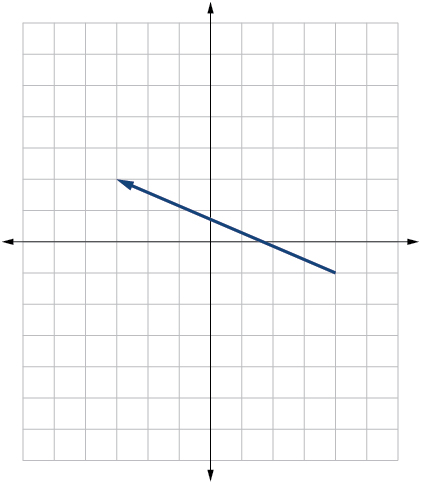# 8.8 Vectors  (Page 8/22)

 Page 8 / 22$〈4,1〉$

Given initial point $\text{\hspace{0.17em}}{P}_{1}=\left(2,1\right)\text{\hspace{0.17em}}$ and terminal point $\text{\hspace{0.17em}}{P}_{2}=\left(-1,2\right),\text{\hspace{0.17em}}$ write the vector $\text{\hspace{0.17em}}v\text{\hspace{0.17em}}$ in terms of $\text{\hspace{0.17em}}i\text{\hspace{0.17em}}$ and $\text{\hspace{0.17em}}j,\text{\hspace{0.17em}}$ then draw the vector on the graph.

Given initial point $\text{\hspace{0.17em}}{P}_{1}=\left(4,-1\right)\text{\hspace{0.17em}}$ and terminal point $\text{\hspace{0.17em}}{P}_{2}=\left(-3,2\right),\text{\hspace{0.17em}}$ write the vector $\text{\hspace{0.17em}}v\text{\hspace{0.17em}}$ in terms of $\text{\hspace{0.17em}}i\text{\hspace{0.17em}}$ and $\text{\hspace{0.17em}}j.\text{\hspace{0.17em}}$ Draw the points and the vector on the graph.

$v=-7i+3j$Given initial point $\text{\hspace{0.17em}}{P}_{1}=\left(3,3\right)\text{\hspace{0.17em}}$ and terminal point $\text{\hspace{0.17em}}{P}_{2}=\left(-3,3\right),\text{\hspace{0.17em}}$ write the vector $\text{\hspace{0.17em}}v\text{\hspace{0.17em}}$ in terms of $\text{\hspace{0.17em}}i\text{\hspace{0.17em}}$ and $\text{\hspace{0.17em}}j.\text{\hspace{0.17em}}$ Draw the points and the vector on the graph.

## Extensions

For the following exercises, use the given magnitude and direction in standard position, write the vector in component form.

$|v|=6,\theta =45°$

$3\sqrt{2}i+3\sqrt{2}j$

$|v|=8,\theta =220°$

$|v|=2,\theta =300°$

$i-\sqrt{3}j$

$|v|=5,\theta =135°$

A 60-pound box is resting on a ramp that is inclined 12°. Rounding to the nearest tenth,

1. Find the magnitude of the normal (perpendicular) component of the force.
2. Find the magnitude of the component of the force that is parallel to the ramp.

a. 58.7; b. 12.5

A 25-pound box is resting on a ramp that is inclined 8°. Rounding to the nearest tenth,

1. Find the magnitude of the normal (perpendicular) component of the force.
2. Find the magnitude of the component of the force that is parallel to the ramp.

Find the magnitude of the horizontal and vertical components of a vector with magnitude 8 pounds pointed in a direction of 27° above the horizontal. Round to the nearest hundredth.

$x=7.13\text{\hspace{0.17em}}$ pounds, $\text{\hspace{0.17em}}y=3.63\text{\hspace{0.17em}}$ pounds

Find the magnitude of the horizontal and vertical components of the vector with magnitude 4 pounds pointed in a direction of 127° above the horizontal. Round to the nearest hundredth.

Find the magnitude of the horizontal and vertical components of a vector with magnitude 5 pounds pointed in a direction of 55° above the horizontal. Round to the nearest hundredth.

$x=2.87\text{\hspace{0.17em}}$ pounds, $\text{\hspace{0.17em}}y=4.10\text{\hspace{0.17em}}$ pounds

Find the magnitude of the horizontal and vertical components of the vector with magnitude 1 pound pointed in a direction of 8° above the horizontal. Round to the nearest hundredth.

## Real-world applications

A woman leaves home and walks 3 miles west, then 2 miles southwest. How far from home is she, and in what direction must she walk to head directly home?

4.635 miles, 17.764° N of E

A boat leaves the marina and sails 6 miles north, then 2 miles northeast. How far from the marina is the boat, and in what direction must it sail to head directly back to the marina?

A man starts walking from home and walks 4 miles east, 2 miles southeast, 5 miles south, 4 miles southwest, and 2 miles east. How far has he walked? If he walked straight home, how far would he have to walk?

17 miles. 10.318 miles

A woman starts walking from home and walks 4 miles east, 7 miles southeast, 6 miles south, 5 miles southwest, and 3 miles east. How far has she walked? If she walked straight home, how far would she have to walk?

A man starts walking from home and walks 3 miles at 20° north of west, then 5 miles at 10° west of south, then 4 miles at 15° north of east. If he walked straight home, how far would he have to the walk, and in what direction?

Distance: 2.868. Direction: 86.474° North of West, or 3.526° West of North

a colony of bacteria is growing exponentially doubling in size every 100 minutes. how much minutes will it take for the colony of bacteria to triple in size
what is the importance knowing the graph of circular functions?
can get some help basic precalculus
What do you need help with?
Andrew
how to convert general to standard form with not perfect trinomial
can get some help inverse function
ismail
Rectangle coordinate
how to find for x
it depends on the equation
Robert
whats a domain
The domain of a function is the set of all input on which the function is defined. For example all real numbers are the Domain of any Polynomial function.
Spiro
foci (–7,–17) and (–7,17), the absolute value of the differenceof the distances of any point from the foci is 24.
difference between calculus and pre calculus?
give me an example of a problem so that I can practice answering
x³+y³+z³=42
Robert
dont forget the cube in each variable ;)
Robert
of she solves that, well ... then she has a lot of computational force under her command ....
Walter
what is a function?
I want to learn about the law of exponent
explain this
what is functions?
A mathematical relation such that every input has only one out.
Spiro
yes..it is a relationo of orders pairs of sets one or more input that leads to a exactly one output.
Mubita
Is a rule that assigns to each element X in a set A exactly one element, called F(x), in a set B.
RichieRich
If the plane intersects the cone (either above or below) horizontally, what figure will be created?ByByBy Michael SagBy Stephanie RedfernBy Brooke DelaneyBy Ryan LoweBy RhodesBy OpenStaxBy RhodesBy Brooke DelaneyByBy OpenStax